Engineering ToolBox - Resources, Tools and Basic Information for Engineering and Design of Technical Applications!

## Heat transfer due to emission of electromagnetic waves is known as thermal radiation.

Heat transfer through radiation takes place in form of electromagnetic waves mainly in the infrared region. Radiation emitted by a body is a consequence of thermal agitation of its composing molecules. Radiation heat transfer can be described by reference to the 'black body'.

### The Black Body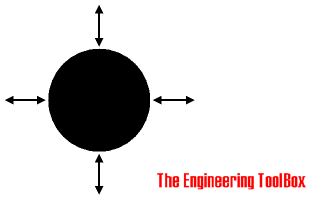The black body is defined as a body that absorbs all radiation that falls on its surface. Actual black bodies don't exist in nature - though its characteristics are approximated by a hole in a box filled with highly absorptive material. The emission spectrum of such a black body was first fully described by Max Planck.

A black body is a hypothetical body that completely absorbs all wavelengths of thermal radiation incident on it. Such bodies do not reflect light, and therefore appear black if their temperatures are low enough so as not to be self-luminous. All black bodies heated to a given temperature emit thermal radiation.

The radiation energy per unit time from a black body is proportional to the fourth power of the absolute temperature and can be expressed with Stefan-Boltzmann Law as

q = σ T4 A                                       (1)

where

q = heat transfer per unit time (W)

σ = 5.6703 10-8 (W/m2K4) - The Stefan-Boltzmann Constant

T = absolute temperature in kelvins (K)

A = area of the emitting body (m2)

#### The Stefan-Boltzmann Constant in Imperial Units

σ = 5.6703 10-8 (W/m2K4)

= 1.714 10-9 ( Btu/(h ft2 oR4) )

=  1.19 10-11 ( Btu/(h in2 oR4) )

###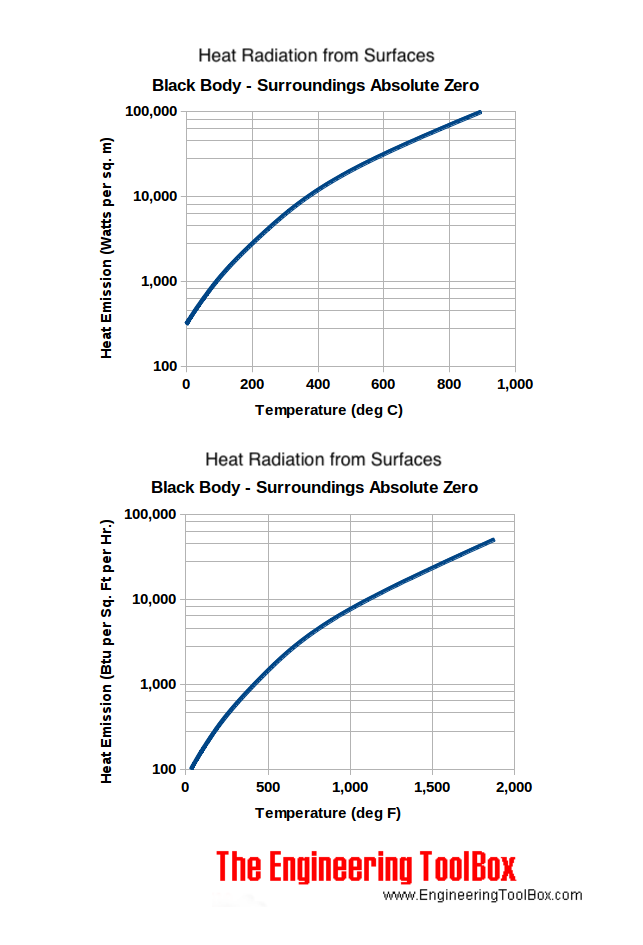Example - Heat Radiation from the surface of the Sun

If the surface temperature of the sun is 5800 K and if we assume that the sun can be regarded as a black body the radiation energy per unit area can be expressed by modifying (1) to

q / A = σ T4

= (5.6703 10-8 W/m2K4) (5800 K)4

= 6.42 107 (W/m2

### Gray Bodies and Emissivity Coefficients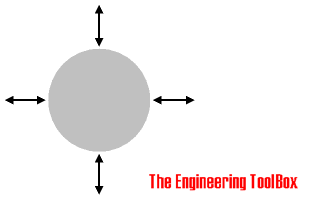For objects other than ideal black bodies ('gray bodies') the Stefan-Boltzmann Law can be expressed as

q = ε σ T4 A                                    (2)

where

ε = emissivity coefficient of the object (one - 1 - for a black body)

For the gray body the incident radiation (also called irradiation) is partly reflected, absorbed or transmitted.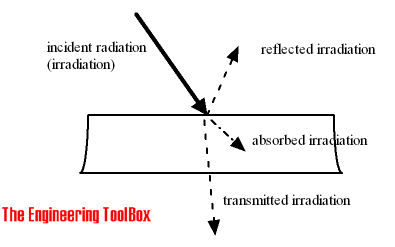The emissivity coefficient is in the range 0 < ε < 1, depending on the type of material and the temperature of the surface.

If an hot object is radiating energy to its cooler surroundings the net radiation heat loss rate can be expressed as

q = ε σ (Th4 - Tc4) Ah                                     (3)

where

Th = hot body absolute temperature (K)

Tc = cold surroundings absolute temperature (K)

Ah = area of the hot object  (m2)

Heat loss from a heated surface to unheated surroundings with mean radiant temperatures are indicated in the chart below.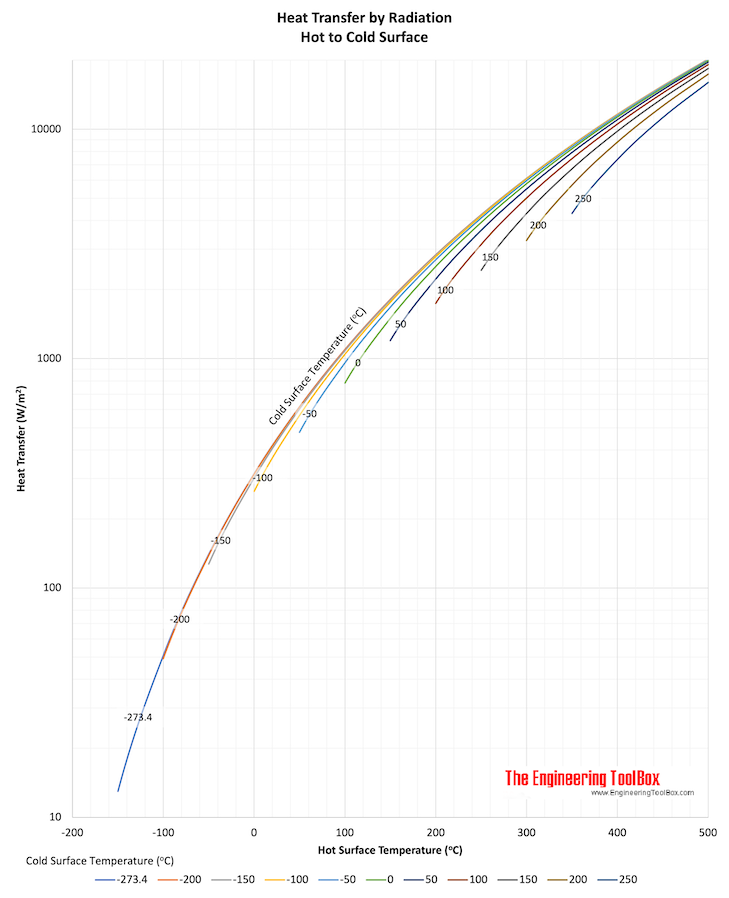This calculator is based on equation (3) and can be used to calculate the heat radiation from a warm object to colder surroundings.

Note that the input temperatures are in degrees Celsius.

ε - emissivity coefficient

th - object hot temperature (oC)

tc - surroundings cold temperature (oC)

Ac - object area (m2)

### Lambert's cosine law

Heat emission from a surface in an angle β can be expressed with Lambert's cosine law as

qβ = q cos β                                         (4)

where

qβ = heat emission in angle β

q = heat emission from the surface

β = angle

## Related Topics

• Heat Loss and Insulation - Heat loss from pipes, tubes and tanks - with and without insulation - foam, fiberglass, rockwool and more.
• Insulation - Heat transfer and heat loss from buildings and technical applications - heat transfer coefficients and insulation methods to reduce energy consumption.
• Heat Loss and Insulation - Steam and condensate pipes - heat loss uninsulated and insulated pipes, insulation thickness and more.
• Thermodynamics - Work, heat and energy systems.

## Engineering ToolBox - SketchUp Extension - Online 3D modeling!

Add standard and customized parametric components - like flange beams, lumbers, piping, stairs and more - to your Sketchup model with the Engineering ToolBox - SketchUp Extension - enabled for use with the amazing, fun and free SketchUp Make and SketchUp Pro .Add the Engineering ToolBox extension to your SketchUp from the SketchUp Pro Sketchup Extension Warehouse!

Translate

## Privacy

We don't collect information from our users. Only emails and answers are saved in our archive. Cookies are only used in the browser to improve user experience.

Some of our calculators and applications let you save application data to your local computer. These applications will - due to browser restrictions - send data between your browser and our server. We don't save this data.

## Citation

• Engineering ToolBox, (2003). Radiation Heat Transfer. [online] Available at: https://www.engineeringtoolbox.com/radiation-heat-transfer-d_431.html [Accessed Day Mo. Year].

Modify access date.

. .

#### Scientific Online Calculator6 24